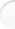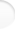Structure of Magic and Semi-Magic Squares, Methods and Tools for Enumeration by Francis Gaspalou1. Subject

You will find here methods and tools for computing sets of magic or semi-magic squares, and even sets of magic cubes.

With a computer, you can easily calculate the exact number of magic or semi-magic squares up to order 4.

It is a little harder to compute sets of a larger order, like the exact number of:
• magic squares of order 5,
• symmetrical pandiagonal squares of order 7,
• ...

For such tasks, you reach the limits of the existing personal computers and so you need methods and tools to reduce computation time, otherwise your computer will work for many days or even for many weeks! Naturally, you need an up-to-date computer, a good computation algorithm and a good programming language. But the best way to reduce computation time is by defining the number of equivalent occurrences or variations of a given square.

For example, for the 7 040 magic squares of order 4, it is well known that you need only compute 880 different squares if you consider the 8 squares given by rotations and reflections as the same square. But you can do better! It is less well known that you need only compute 220 different squares. In other words, a given magic square of order 4 has more than 8 variations, it has 32, and these are 32 variations of the same square. These 32 transformations of the same square have a group structure. I give in Appendix 3, § 1 the exact definition of this group of order 32.

In mathematical terms, the transformations on magic or semi-magic squares have a group structure. Therefore, when computing a set of squares, the first task is to define the dimension of this group of transformations.

There is not a lot of literature in this domain and my main contribution is synthesizing various scattered results. On this subject, I think that more synthesis is needed rather than new results. However, I found in fact some original results.

Caution! By method, I don't mean methods of construction, like de la HIRE's method or de la LOUBERE's method, but methods for reducing computation time, like using a group of transformations.
The fundamental question for me is: if you have a given square, how many other equivalent squares can you derive? You consider all the induced squares as the same square. The list of squares can then be reduced to a list of "elementary squares" or "essentially different squares".

 2. Application Top of the pageMethods and tools for deriving equivalent squares from a given square may be classified into two main categories:

• the transformation method which consists in applying an operator (like a rotation or a symmetry or more generally a transformation defined as a matrix of order n) on the position of the cells;

• the permutation method which consists in applying an operator on the contents of the cells (and not on their position).

The two methods may be combined.

The intermediate square method which consists in decomposing a square into two squares (capital letters and lower-case letters) is useful for representing some sets of squares.

Then the enumeration of squares (general or reduced by the methods indicated) is done with a computer. The principle of the program is very simple: you have to nest loops. The choice of the programming language is very important for performance.
The large number of cases to examine is the problem. Thus you only compute:
• squares with particular conditions like symmetrical, pandiagonal squares, ... which are in fact families with a large population
• orthogonal squares to a given lower-case square (this method seems original)
• squares with random values for some parameters (this method allows estimating the number of magic squares for high orders and was invented by Walter TRUMP: see his site http://www.trump.de/magic-squares)
• ...

All these methods may be used for magic squares as well as for semi-magic squares, and even for magic cubes.

I concentrate on the transformation method as it is the most important.

Note: my paper can be analysed as the application of the group theory to magic squares. The subject theoretically requires a fairly good level in mathematics (group theory, set theory, vector spaces) but it is not compulsory! I try to make these notions simple enough for an amateur. My paper is addressed both to non professionals trying to understand the subject and to specialists in the field.

 3. Conclusion Top of the pageWith today's powerful personal home computers, anyone may have fun with magic squares (whereas it was reserved for a happy few 25 years ago). However, more methods and tools are required to reduce computation time, to explain results and to understand the intimate structure of magic squares. The purpose of this paper is to provide useful guidance in this domain.